### Home > CALC > Chapter 12 > Lesson 12.1.3 > Problem12-40

12-40.
1. Multiple Choice: To find the area of the region in the first quadrant outside the circle r = 4 cos θ and inside the lemniscate r2 = 8 sin 2θ , use the integral: Homework Help ✎

1.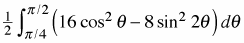2.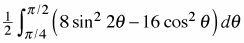3.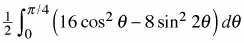4.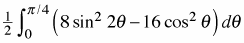5.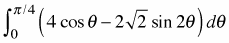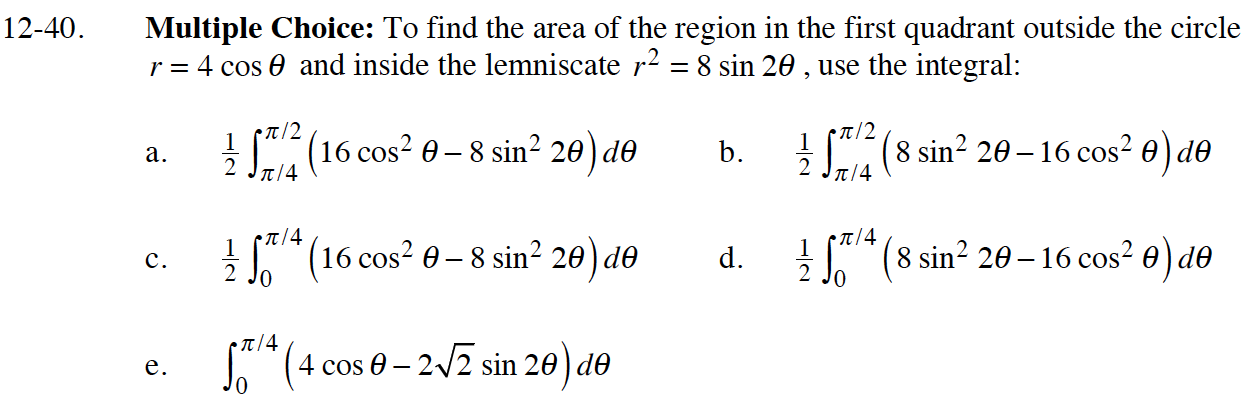Determine where the functions intersect for the bounds of integration. Remember, this is only in the first quadrant.

$16\cos^2(\theta)=8\sin(2\theta)$

$16\cos^2(\theta)=16\sin(\theta)\cos(\theta)$

Graph the functions to determine which is the "upper" function and which is the "lower" function.

Use the area inside polar curves formula: